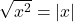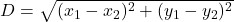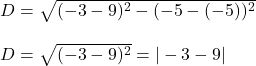## Explain why |−3| + |9| represents the distance between the points (−3Explain why |−3| + |9| represents the distance between the points (−3,

Question

Explain why |−3| + |9| represents the distance between the points (−3Explain why |−3| + |9| represents the distance between the points (−3, −5) and (9, −5)., −5) and (9, −5).

in progress 0
4 months 2021-07-26T02:09:24+00:00 1 Answers 4 views 0

Here we need to use:The distance between two points (x₁, y₁) and (x₂, y₂) is given by:Then the distance between:

(-3, -5) and (9, -5) is just:because both numbers inside the absolute value, we can rewrite it as:

|-3 – 9| = |-3| + |-9| = |-3| + |9| = 12

So, by finding the distance between  (−3, −5) and (9, −5), we got the given expression, in this way we prove that the given expression represents the distance between these points.